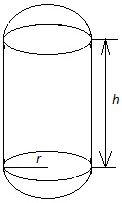Courses
Courses for Kids
Free study material
Free LIVE classes
MoreLIVE
Join Vedantu’s FREE Mastercalss

# A solid composed of a cylinder with hemispherical ends on both sides. The radius and height of the cylinder are 20 cm and 30 cm respectively. Find the total surface area of the solid. Take $\pi =3\cdot 14$.Verified
360k+ views
Hint: First assume radius of cylinder = radius of hemisphere = $r$. Let height of the cylinder $=h$. Then we should use the formulas, Lateral surface area (L.S.A.) of cylinder $=2\pi rh$, Lateral surface area (L.S.A.) of hemisphere $=2\pi {{r}^{2}}$, to calculate the area we need in the question.

Let the height of the cylinder is $h$. Since the radii of the hemisphere and cylinder are equal, we assume their radius as $r$.
We have been given that $r=20\text{ cm}$ and $h=30\text{ cm}$.
Now, we have to imagine the figure when a solid cylinder has hemispherical ends on both its sides. We will come to the conclusion that only the lateral surface area of the cylinder and hemisphere are visible as both the circular bases of the cylinder overlap with the base of the hemisphere.Therefore, the total surface area of the solid we need to calculate is the sum of lateral surface area of the cylinder and that of the hemisphere.
Required T.S.A. of solid $=$ L.S.A. of cylinder $+$ L.S.A. of hemisphere.
Required T.S.A. of solid $=2\pi rh+2\pi {{r}^{2}}...................................(1)$
Substituting the given values of $r$ and $h$ in equation $(1)$, we get;
\begin{align} & \text{Required T}\text{.S}\text{.A}\text{. of solid}=2\times 3.14\times 20\times 30+4\times 3.14\times {{20}^{2}} \\ & \text{ }=3768+5024 \\ & \text{ }=8792\text{ c}{{\text{m}}^{2}} \\ \end{align}

Note: We have to imagine the diagram properly or it is better to draw it somewhere, otherwise one may get confused. Basic formulas of surface areas of different shapes must also be remembered. We have to make sure that all the lengths were taken in the same units to find the area.
Last updated date: 23rd Sep 2023
Total views: 360k
Views today: 7.60k